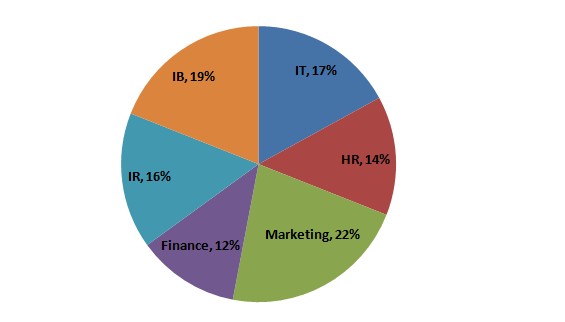Directions : Study the pie-chart carefully to answer the questions that follow.

Total number of students are 8000Data Provided: Percentage Wise Break up of Students in terms of Specialization in MBA

Question 1: What is the total number of students having specialization in IR, Marketing and IT?

(a) 4640
(b) 4080
(c) 4260
(d) 4400

Question 2: Students having IB as specialization forms approximately what percent of students having Marketing as specialization?

(a) 116%
(b) 86%
(c) 124%
(d) 74%

Question 3: What is the total number of students having IB as specialization?

(a) 1520
(b) 1280
(c) 1360
(d) 1120

Question 4: What is the respective ratio of the students having Finance as specialization to that of students having HR as specialization?

(a) 11 : 19
(b) 18 : 13
(c) 6 : 7
(d) 12 : 21

Answer 1: (d) The number of students having specialization in IR, Marketing and IT is 55% of the total students.

Hence, the required percentage = (55/100) x 8000 = 4400

The correct option is (d)

There are 19% students who have specialization in IB and 22% students have specialization in Marketing.

So, the required percentage =(19/22) x 100 = 86.36%

The correct option is (b)

Total number of students with IB as specialization = 19% of 8000 = 1520.

The correct option is (a)